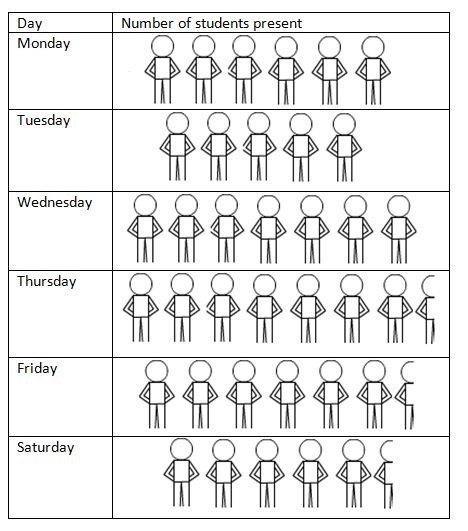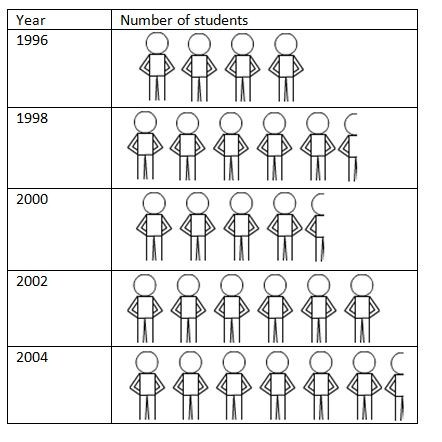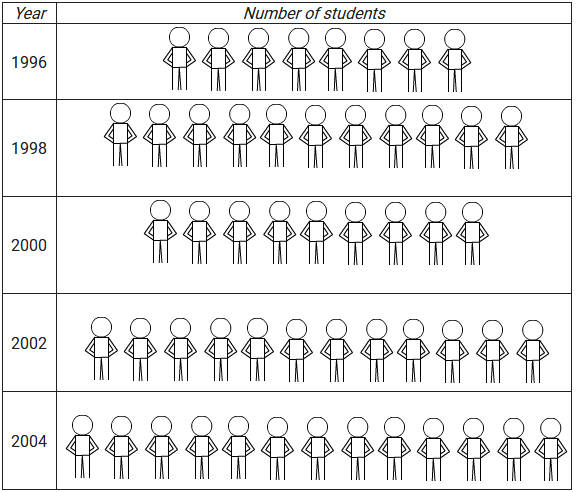×#### Thank you for registering.

One of our academic counsellors will contact you within 1 working day.

Click to Chat

1800-1023-196

+91-120-4616500

CART 0

• 0

MY CART (5)

Use Coupon: CART20 and get 20% off on all online Study Material

ITEM
DETAILS
MRP
DISCOUNT
FINAL PRICE
Total Price: Rs.

There are no items in this cart.
Continue Shopping• Complete JEE Main/Advanced Course and Test Series
• OFFERED PRICE: Rs. 15,900
• View Details

```Chapter 22 Data Handling II (Pictographs) Exercise 22.2

Question: 1

The following are the details or the number of students in a class of 30 students presents a week.

Day
Number of students present

Monday
24

Tuesday
20

Wednesday
28

Thursday
30

Friday
26

Saturday
22

Represent the above data by a pictograph.

Solution:

Let an icon of a student represent 4 students. Then, the number of icons for each day is as follows:

Day
Number of students present

Monday
24 / 4 = 6

Tuesday
20 / 4 = 5

Wednesday
28 / 4 = 7

Thursday
30 / 4 = 7.5

Friday
26 / 4 = 6.5

Saturday
22 / 4 = 5.5

The pictograph representing the above data is as follows:Question: 2

Total number of students of a school in different years is shown in the following table:

Year
Number of students

1996
400

1998
550

2000
450

2002
600

2006
650

Represent the above data by a pictograph.

(a) Prepare a pictograph of students using one symbol an icon of a student to represent students and answer the following questions:

(i) How many symbols represent total number of students in the year 2002?

(ii) How many symbols represent total number of students for the year 1998?

(b) Prepare another pictograph of students using any other symbol each represents students. Which pictograph do you find more informative?

Solution:

(a) Let one icon represent 100 students.

Then, the numbers of icons for different years are as follows:

Year
Number of students

1996
400 / 100 = 4

1998
550 / 100 = 5.5

2000
450 / 100 = 4.5

2002
600 / 100 = 6

2006
650 / 100 = 6.5

The pictograph representing the above data is as follows:(i) 6 icons represent the total number of students in the year 2002.

(ii) 5 and half icons represent the total number of students in the year 1998.

(b) Let one icon represent 50 students.

Then, the numbers of icons for different years are as follows:

Year
Number of students

1996
400 / 50 = 8

1998
550 / 50 = 11

2000
450 / 50 = 9

2002
600 / 50 = 12

2006
650 / 50 = 13Explanation: in the second pictograph, every icon is complete, therefore more convenient to understand.
```### Course Features

• 728 Video Lectures
• Revision Notes
• Previous Year Papers
• Mind Map
• Study Planner
• NCERT Solutions
• Discussion Forum
• Test paper with Video Solution